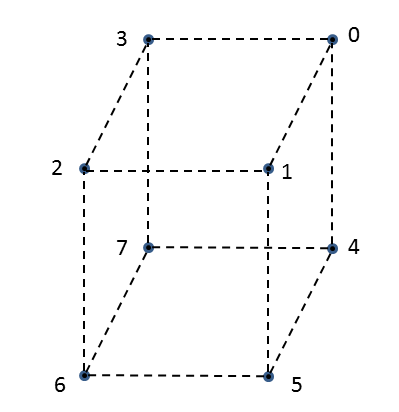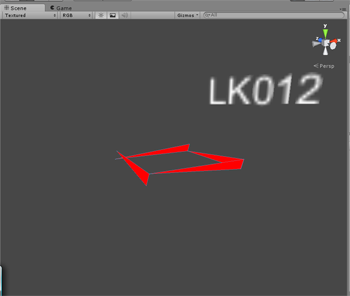# Making a Procedural Cube MeshI’m attempting to make a procedural mesh on a cube. I only want the sides to have the mesh, not the top or bottom.

For the vertices I’m doing the following:

``````// create the vertices array
for ( int ptsIndex = 0; ptsIndex < numVerts; ptsIndex++ ){
// x, y, z are generated here ...
// blah, blah, blah

switch ( ptsIndex % 4 ){
case 0:
verts[ ptsIndex ] = new Vector3( x + deltaValue, y, z - deltaValue );
break;
case 1:
verts[ ptsIndex ] = new Vector3( x + deltaValue, y, z + deltaValue );
break;
case 2:
verts[ ptsIndex ] = new Vector3( x - deltaValue, y, z + deltaValue );
break;
case 3:
verts[ ptsIndex ] = new Vector3( x - deltaValue, y, z - deltaValue );
break;
}
}
``````

Then I create my triangle array:

``````mesh.triangles = new int[]{ 0, 1, 5,
0, 4, 5,
1, 2, 6,
1, 5, 6,
2, 3, 7,
2, 6, 7,
3, 0, 4,
3, 7, 4 };
``````

And finally assign everything:

``````	mesh.vertices = verts;
mesh.triangles = tris;

mesh.RecalculateNormals();
mesh.RecalculateBounds();
mesh.Optimize();
``````

Here is a screen shot of what I’m getting:If any of you have any ideas on what I’m doing wrong I would love to hear it!

Thanks!

Jim

It’s called Vertex Winding. The clock-wise counter-clockwise order of your verts will determine which direction is the front, in the absence of providing a normal vector.